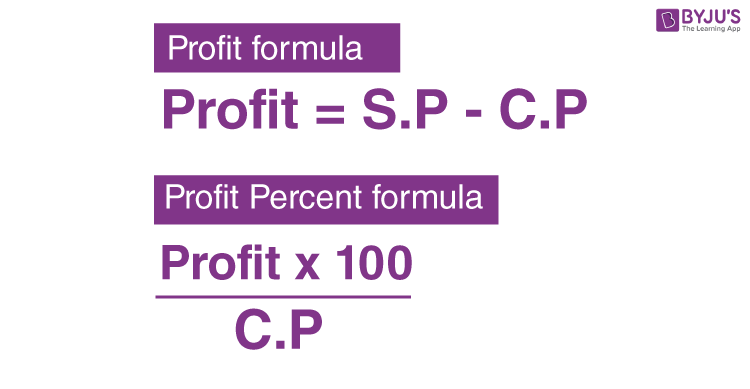# Profit Formula

Profit formula is used to know how much profit has been made by selling a particular product. Formula for profit is majorly used for business and financial transactions. Profit arises when the selling price of any product sold is greater than the cost price (that is the price at which the product was originally bought). It should be noted that the profit and loss as a percentage is generally used to depict how much profit or loss a trader gets from a particular deal.## Formulas to Calculate Profit

The Profit Formulas are given as:

Formula for Profit Profit = S.P – C.P.
Formula for Profit Percentage Profit Percent Formula =
$$\begin{array}{l}\frac{Profit\times100}{C.P.}\end{array}$$
Gross Profit Formula Gross Profit = Revenue – Cost of Goods Sold
Profit Margin Formula Profit Margin =
$$\begin{array}{l}\frac{Total\;Income}{Net\;Sales} \times 100\end{array}$$
Gross Profit Margin Formula Gross Profit Margin =
$$\begin{array}{l}\frac{Gross\;Profit}{Net\;Sales}\times 100\end{array}$$

### Notation Used in Profit Formula

• S.P. = Selling Price i.e. the cost at which the product is sold
• C.P. = Cost price i.e. the cost at which the product is originally bought

### Example Question Using the Formula for Profit

Question: A shopkeeper buys watches in bulk for Rs. 20 each. He sells them for Rs. 45 each. Calculate the profit and the profit percentage.

Solution:

Given,

Selling price of the watch = Rs. 45

Cost price of the watch = Rs. 20

Now, Profit = Selling Price – Cost Price

So, profit on the watch = 45 – 20 = Rs. 25

Using the formula for profit percentage,

Profit % = (Profit / C.P.) × 100

So, the profit percentage of the shopkeeper will be (25 / 20) × 100 = 1.25 × 100 = 125%.

It can be said that the shopkeeper made a profit of Rs. 25 from each watch with a profit percentage of 125%.

Stay tuned with BYJU’S for more maths formulas and concepts for different classes. Also, get various shortcut tricks, preparation tips, question papers, sample papers, video lessons, important questions and other study materials at BYJU’S to learn mathematics in a more effective way.